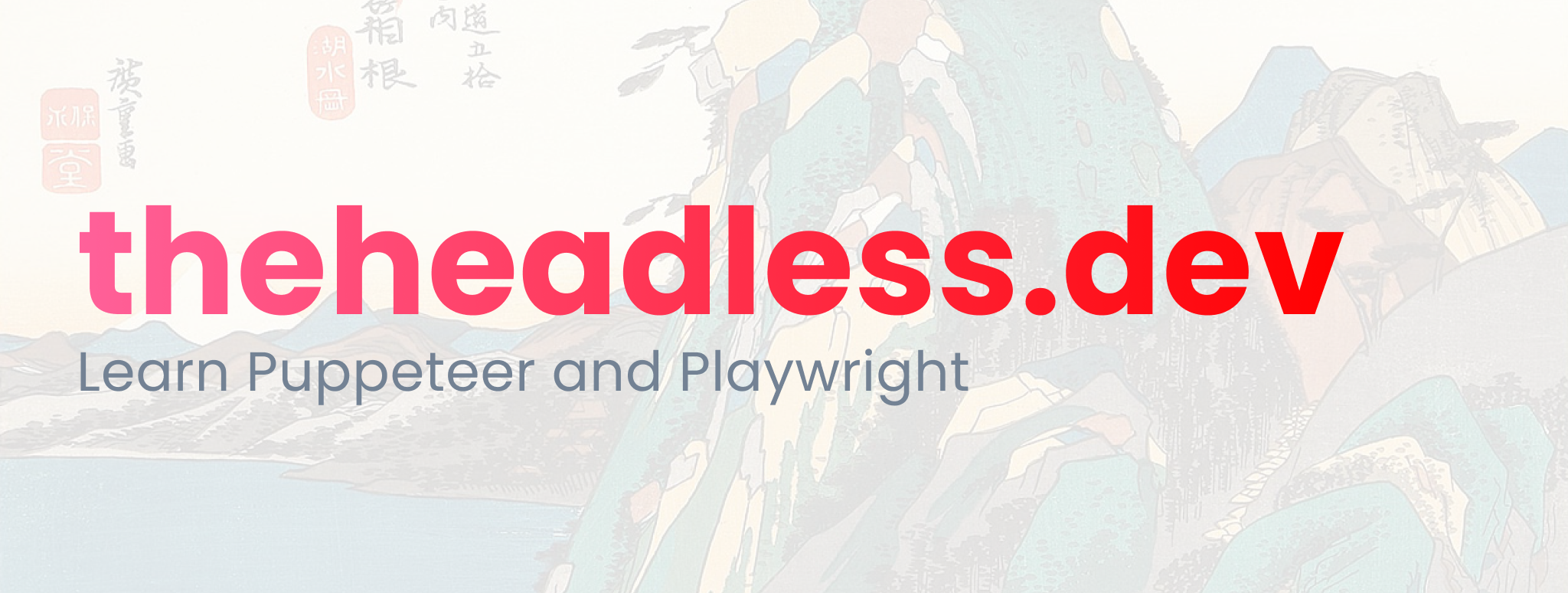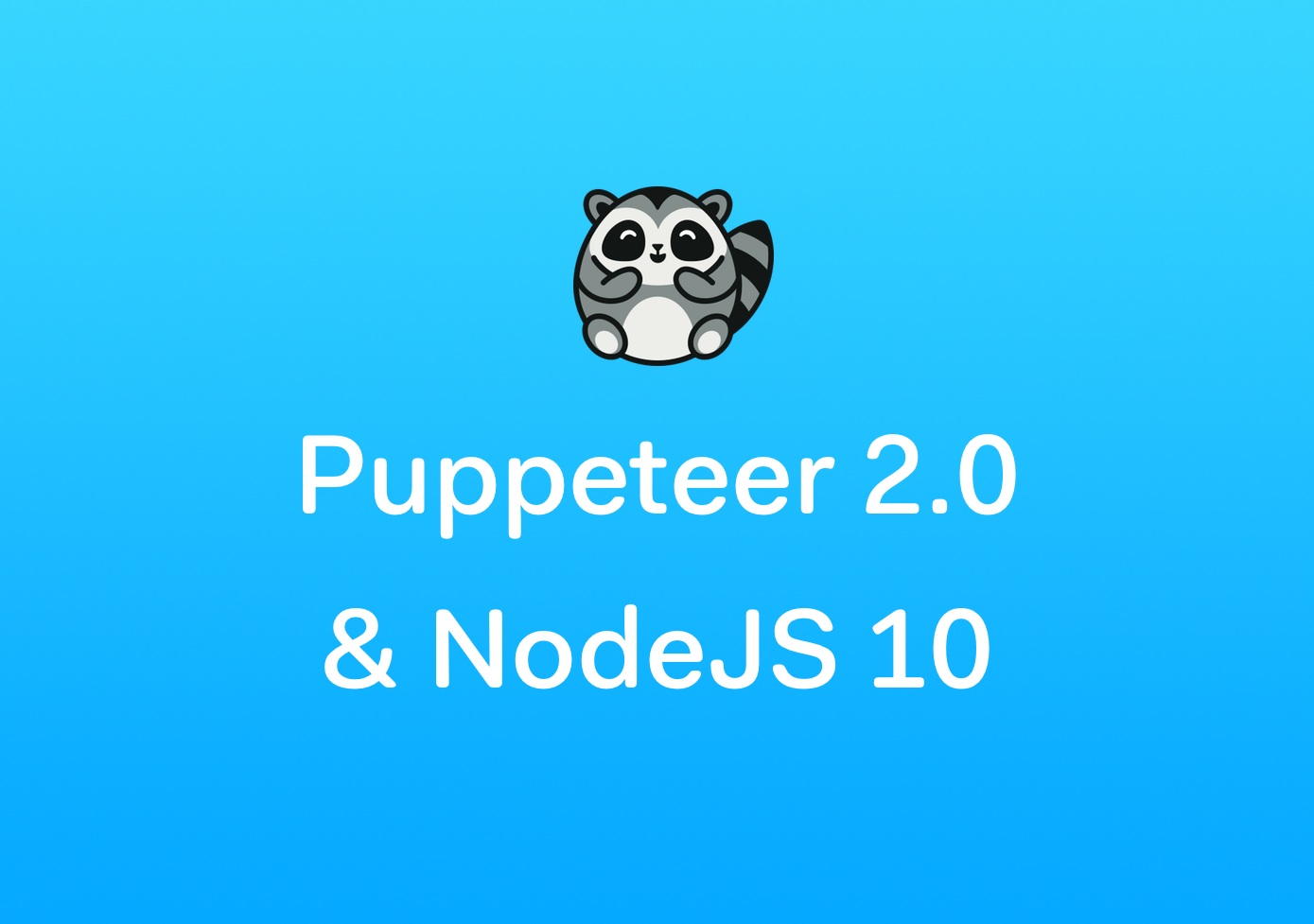# #puppeteer

Bookmark the official Checkly blog for the latest news, tips, tricks, and how-to articles on Checkly's advanced synthetic monitoring platform.## Playwright Explainedself.__wrap_b=(t,n,e)=>{e=e||document.querySelector(`[data-br="\${t}"]`);let a=e.parentElement,r=R=>e.style.maxWidth=R+"px";e.style.maxWidth="";let o=a.clientWidth,c=a.clientHeight,i=o/2-.25,l=o+.5,u;if(o){for(;i+1<l;)u=Math.round((i+l)/2),r(u),a.clientHeight===c?l=u:i=u;r(l*n+o*(1-n))}e.__wrap_o||(typeof ResizeObserver!="undefined"?(e.__wrap_o=new ResizeObserver(()=>{self.__wrap_b(0,+e.dataset.brr,e)})).observe(a):process.env.NODE_ENV==="development"&&console.warn("The browser you are using does not support the ResizeObserver API. Please consider add polyfill for this API to avoid potential layout shifts or upgrade your browser. Read more: https://github.com/shuding/react-wrap-balancer#browser-support-information"))};self.__wrap_b(":R1j566:",1)Jeff James## Cypress vs Selenium vs Playwright vs Puppeteer speed comparisonself.__wrap_b=(t,n,e)=>{e=e||document.querySelector(`[data-br="\${t}"]`);let a=e.parentElement,r=R=>e.style.maxWidth=R+"px";e.style.maxWidth="";let o=a.clientWidth,c=a.clientHeight,i=o/2-.25,l=o+.5,u;if(o){for(;i+1<l;)u=Math.round((i+l)/2),r(u),a.clientHeight===c?l=u:i=u;r(l*n+o*(1-n))}e.__wrap_o||(typeof ResizeObserver!="undefined"?(e.__wrap_o=new ResizeObserver(()=>{self.__wrap_b(0,+e.dataset.brr,e)})).observe(a):process.env.NODE_ENV==="development"&&console.warn("The browser you are using does not support the ResizeObserver API. Please consider add polyfill for this API to avoid potential layout shifts or upgrade your browser. Read more: https://github.com/shuding/react-wrap-balancer#browser-support-information"))};self.__wrap_b(":R1l566:",1)Giovanni Rago## Puppeteer vs Selenium vs Playwright, a speed comparisonself.__wrap_b=(t,n,e)=>{e=e||document.querySelector(`[data-br="\${t}"]`);let a=e.parentElement,r=R=>e.style.maxWidth=R+"px";e.style.maxWidth="";let o=a.clientWidth,c=a.clientHeight,i=o/2-.25,l=o+.5,u;if(o){for(;i+1<l;)u=Math.round((i+l)/2),r(u),a.clientHeight===c?l=u:i=u;r(l*n+o*(1-n))}e.__wrap_o||(typeof ResizeObserver!="undefined"?(e.__wrap_o=new ResizeObserver(()=>{self.__wrap_b(0,+e.dataset.brr,e)})).observe(a):process.env.NODE_ENV==="development"&&console.warn("The browser you are using does not support the ResizeObserver API. Please consider add polyfill for this API to avoid potential layout shifts or upgrade your browser. Read more: https://github.com/shuding/react-wrap-balancer#browser-support-information"))};self.__wrap_b(":R1n566:",1)Giovanni Rago## Active monitoring with Playwright on Checkly 🎭self.__wrap_b=(t,n,e)=>{e=e||document.querySelector(`[data-br="\${t}"]`);let a=e.parentElement,r=R=>e.style.maxWidth=R+"px";e.style.maxWidth="";let o=a.clientWidth,c=a.clientHeight,i=o/2-.25,l=o+.5,u;if(o){for(;i+1<l;)u=Math.round((i+l)/2),r(u),a.clientHeight===c?l=u:i=u;r(l*n+o*(1-n))}e.__wrap_o||(typeof ResizeObserver!="undefined"?(e.__wrap_o=new ResizeObserver(()=>{self.__wrap_b(0,+e.dataset.brr,e)})).observe(a):process.env.NODE_ENV==="development"&&console.warn("The browser you are using does not support the ResizeObserver API. Please consider add polyfill for this API to avoid potential layout shifts or upgrade your browser. Read more: https://github.com/shuding/react-wrap-balancer#browser-support-information"))};self.__wrap_b(":R1p566:",1)Jan GrzesikBatuhan Icoz## Headless browser automation guide - Writing theheadless.devself.__wrap_b=(t,n,e)=>{e=e||document.querySelector(`[data-br="\${t}"]`);let a=e.parentElement,r=R=>e.style.maxWidth=R+"px";e.style.maxWidth="";let o=a.clientWidth,c=a.clientHeight,i=o/2-.25,l=o+.5,u;if(o){for(;i+1<l;)u=Math.round((i+l)/2),r(u),a.clientHeight===c?l=u:i=u;r(l*n+o*(1-n))}e.__wrap_o||(typeof ResizeObserver!="undefined"?(e.__wrap_o=new ResizeObserver(()=>{self.__wrap_b(0,+e.dataset.brr,e)})).observe(a):process.env.NODE_ENV==="development"&&console.warn("The browser you are using does not support the ResizeObserver API. Please consider add polyfill for this API to avoid potential layout shifts or upgrade your browser. Read more: https://github.com/shuding/react-wrap-balancer#browser-support-information"))};self.__wrap_b(":R1r566:",1)Giovanni Rago## Changelog: Puppeteer & Chromium updateself.__wrap_b=(t,n,e)=>{e=e||document.querySelector(`[data-br="\${t}"]`);let a=e.parentElement,r=R=>e.style.maxWidth=R+"px";e.style.maxWidth="";let o=a.clientWidth,c=a.clientHeight,i=o/2-.25,l=o+.5,u;if(o){for(;i+1<l;)u=Math.round((i+l)/2),r(u),a.clientHeight===c?l=u:i=u;r(l*n+o*(1-n))}e.__wrap_o||(typeof ResizeObserver!="undefined"?(e.__wrap_o=new ResizeObserver(()=>{self.__wrap_b(0,+e.dataset.brr,e)})).observe(a):process.env.NODE_ENV==="development"&&console.warn("The browser you are using does not support the ResizeObserver API. Please consider add polyfill for this API to avoid potential layout shifts or upgrade your browser. Read more: https://github.com/shuding/react-wrap-balancer#browser-support-information"))};self.__wrap_b(":R1t566:",1)Batuhan Icoz